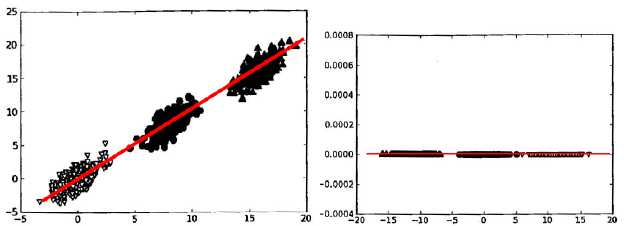# PCA降维

## 概念

PCA的本质就是找一些投影方向，使得数据在这些投影方向上的方差最大，而且这些投影方向是相互正交的。这其实就是找新的正交基的过程，计算原始数据在这些正交基上投影的方差，方差越大，就说明在对应正交基上包含了更多的信息量。可以证明原始数据协方差矩阵的特征值越大，对应的方差越大，在对应的特征向量上投影的信息量就越大。

PCA的全部工作简单点说，就是对原始的空间中顺序地找一组相互正交的坐标轴，第一个轴是使得方差最大的，第二个轴是在与第一个轴正交的平面中使得方差最大的，第三个轴是在与第1、2个轴正交的平面中方差最大的，这样假设在N维空间中，我们可以找到N个这样的坐标轴，我们取前r个去近似这个空间，这样就从一个N维的空间压缩到r维的空间了，但是我们选择的r个坐标轴能够使得空间的压缩使得数据的损失最小。$\frac{\sum_{i=1}^k v_i}{\sum_{i=1}^n v_i}$

$var(x) = \frac{\sum_{i=1}^n (x_i -\bar x)^2}{n-1}$

$cov(x, y) = \frac{\sum_{i=1}^n (x_i -\bar x)(y_i - \bar y)}{n - 1}$

$\sum = \begin{pmatrix} cov(x, x) & cov(x, y) & cov(x, z) \... & cov(y ,y) & cov(y, z) \... & ... & cov(z, z) \ \end{pmatrix}$

• 最近重构性：样本点到这个超平面的距离都足够近；
• 最大可分性：样本点在这个超平面上的投影能尽量分开。

## 计算过程

1. 设有m条n维数据。
2. 将原始数据按列组成n行m列矩阵X
3. 将X的每一行（代表一个属性字段）进行零均值化，即减去这一行的均值
4. 求出协方差矩阵
5. 求出协方差矩阵的特征值及对应的特征向量
6. 将特征向量按对应特征值大小从上到下按行排列成矩阵，取前k行组成矩阵P
7. 即为降维到k维后的数据

PCA计算举例

## 使用Demo

from sklearn.decomposition import PCA
import numpy as np
from sklearn.preprocessing import StandardScaler

x = np.array([[10001, 2, 55], [16020, 4, 11], [12008, 6, 33], [13131, 8, 22]])
# 标准化，可取消
X_scaler = StandardScaler()
x = X_scaler.fit_transform(x)
print(x, "\n")
# PCA
pca = PCA(n_components=0.9)  # 保证降维后的数据保持90%的信息
pca.fit(x)
print(pca.transform(x))
"""

[ 1.48440157 -0.4472136  -1.18321596]
[-0.35938143  0.4472136   0.16903085]
[ 0.15671236  1.34164079 -0.50709255]]

[[ 2.36863319  0.38298087]
[-1.50952734  1.23481789]
[ 0.14360068 -0.58040917]
[-1.00270653 -1.03738959]]
"""

PCA方法参数n_components，如果设置为整数$$x$$,则表示将数据降至$$x$$维，如果是小数，则表明降维后保留的信息量比例。

## 参考链接

PCA降维

(0)
(0)